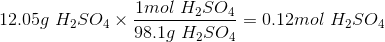# GED Science : Moles

## Example Questions

### Example Question #1 : Chemistry

Atomic mass values: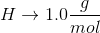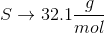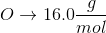Convert the following mass to moles: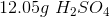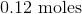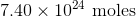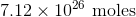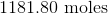Explanation:

To convert grams to moles, use the molecular weight of the molecule. This is found by adding the mass of each atom.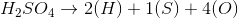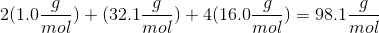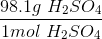Now we can use the molecular mass to convert the given mass in the question to moles. Make sure to align the values so that the units cancel out.